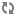﻿ 用等式，领悟人生。终身铭记这道题 ! | 盛飞在线

1 2 3 4 5 6 7 8 9 10 11 12 13 14 15 16 17 18 19 20 21 22 23 24 25 26

Hard work （努力工作）
H+A+R+D+W+O+R+K＝8+1+18+4+23+15+18+11=98%

Knowledge（知识）
K+N+O+W+L+E+D+G+E＝11+14+15+23+12+5+4+7+5=96%

Love（爱情）
L+O+V+E＝12+15+22+5=54%

Luck（好运）
L+U+C+K＝12+21+3+11=47%

Money（金钱）吗? …

ATTITUDE（心态）
A+T+T+I+T+U+D+E＝1+20+20+9+20+21+4+5=100%

Only One Response
Comment (1)Loading ....
•